a
aaa阿伟呀

栈

1) 栈的英文为 (stack)
2) 栈是一 先入后出 (FILO-First In Last Out) 的有序列表
3) (stack) 是限制线性表中元素的插入和删除 只能在线性表的同一端 进行的一种特殊线性表。允许插入和删除的一端，为 变化的一端，称为 栈顶 (Top) ，另一端为 固定的一端，称为 栈底 (Bottom)
4) 根据栈 的定义可知，最先放入栈中元素在栈底，最后放入的元素在栈顶，而删除元素刚好相反，最后放入的元素最先删除，最先放入的元素最后删除

栈的应用场景

1) 程序的调用：在跳往子程序前，会先将下个指令的地址存到堆栈中，直到子程序执行完后再将地址取出，以回到原来的程序中
2) 理递归调用：和子程序的调用类似，只是除了储存下一个指令的地址外，也将参数、区域变量等数据存入堆栈中
3) 达式的转 [ 中缀表达式转后缀表达式 ] ( 实际解决 )
4) 叉树的遍历
5) 形的深度优先 (depth first) 搜索法。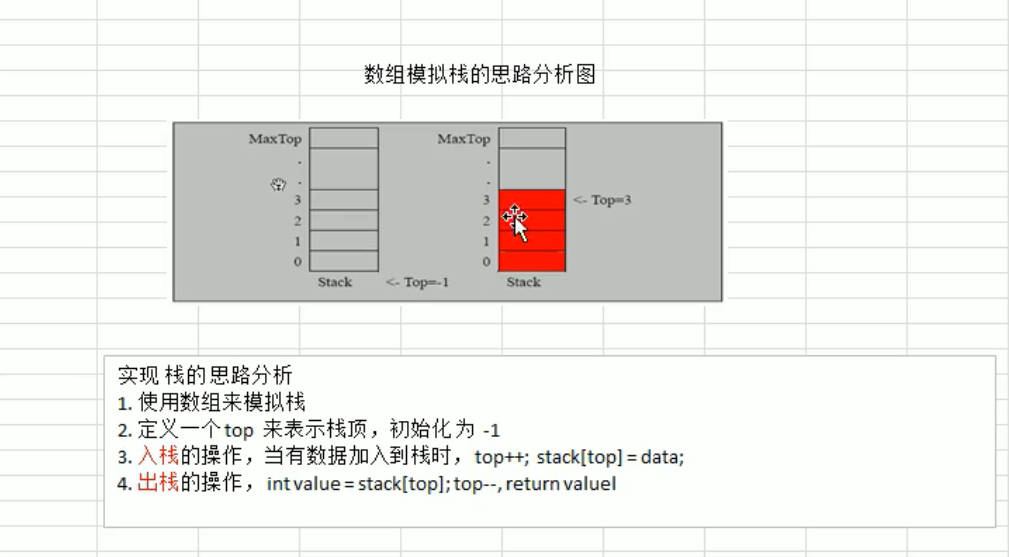栈的代码实现

//定义一个 ArrayStack 表示栈
class ArrayStack {
private int maxSize; // 栈的大小
private int[] stack; // 数组，数组模拟栈，数据就放在该数组
private int top = -1;// top表示栈顶，初始化为-1

//构造器
public ArrayStack(int maxSize) {
this.maxSize = maxSize;
stack = new int[this.maxSize];
}

//栈满
public boolean isFull() {
}

//栈空
public boolean isEmpty() {
}

//入栈
public void push(int value) {
//先判断栈是否满
if(isFull()) {
System.out.println("栈满");
return;
}
top++;
stack[top] = value;
}

//出栈
//将栈顶的数据返回
public int pop() {
//先判断栈是否空
if(isEmpty()) {
//抛出异常
throw new RuntimeException("栈空，没有数据~");
}
int value = stack[top];
top--;
return value;
}

//[遍历栈]， 遍历时，需要从栈顶开始显示数据
public void list() {
if(isEmpty()) {
System.out.println("栈空，没有数据~~");
return;
}
//需要从栈顶开始显示数据
for(int i = top; i >= 0 ; i--) {
System.out.printf("stack[%d]=%d\n", i, stack[i]);
}
}

public static void main(String[] args) {
//先创建一个arraystack对象表示栈
ArrayStack stack =new ArrayStack(4);
String key= "";
boolean loop = true;
Scanner scanner =new Scanner(System.in);
while (loop){
System.out.println("show: 表示显示栈");
System.out.println("exit: 退出程序");
System.out.println("push: 表示添加数据到栈(入栈)");
System.out.println("pop: 表示从栈取出数据(出栈)");
System.out.println("请输入你的选择");
key = scanner.next();
switch (key) {
case "show":
stack.list();
break;
case "push":
System.out.println("请输入一个数");
int value = scanner.nextInt();
stack.push(value);
break;
case "pop":
try {
int res = stack.pop();
System.out.printf("出栈的数据是 %d\n", res);
} catch (Exception e) {
// TODO: handle exception
System.out.println(e.getMessage());
}
break;
case "exit":
scanner.close();
loop = false;
break;
default:
break;
}
}
System.out.println("程序退出了！");
}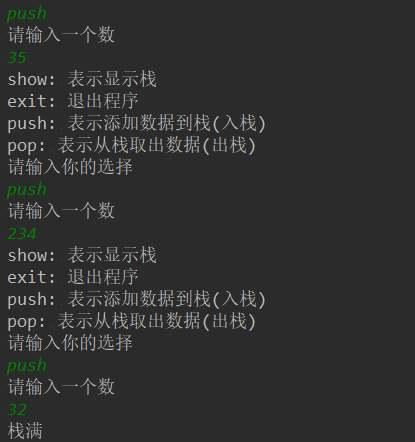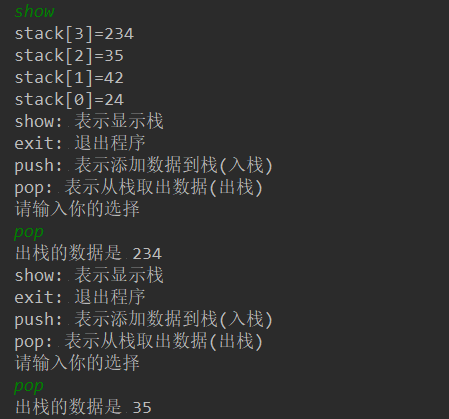递归（Recursion）

1) 打印 问题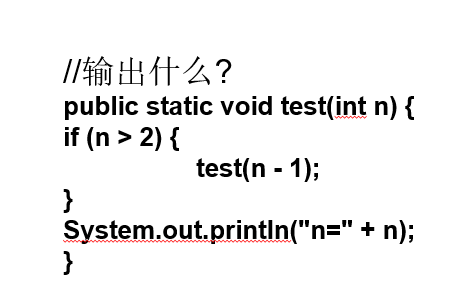2) 阶乘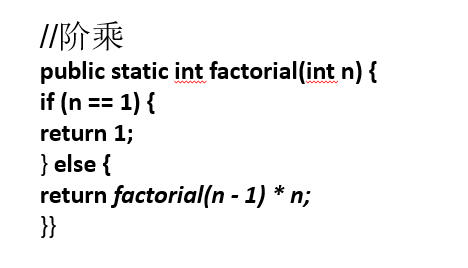递归用于解决什么样的问题

1) 种数学问题如 : 8 后问题 , 汉诺塔 , 阶乘问题 , 迷宫问题 , 球和篮子的问题 (google 编程大赛 )
2) 种算法中也会使用到递归，比如快排，归并排序，二分查找，分治算法等 .
3) 用栈解决的问题 --> 第归代码比较简洁

递归需要遵守的重要规则

1) 行一 方法 ，就创建一个新的受保护的独立空间 ( 空间 )
2) 方法 局部变量是独立的，不会相互影 , 比如 n
3) 果方法中使用的是引用类型变量 ( 比如数组 ) ，就会共享该引用类型的数据 .
4) 归必须向退出递归的条件逼近，否则就是无限递 , 出现 StackOverflowError 死龟了 :)
5) 方法 行完毕，或者遇到 return ，就会返回，遵守谁调用，就将结果返回给谁，同时 方法 行完毕或者返回时， 就执行完毕。
l 递归应用场景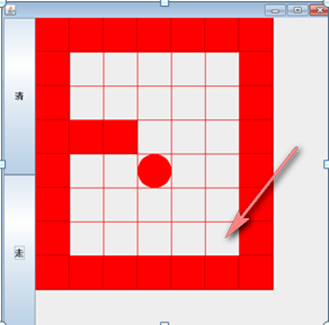2)再得到小球路径时，可以先使用(下右上左)，再改成(下左)看路径是不是有变化

3) 试回溯 现象
4) : 如何求出最短路径 ?

/**
* @author Sun.Mr
* @create 2019-09-17 22:21
*/
public class Migong {
public static void main(String[] args) {
//先创建一个二维数组，模拟迷宫
//地图
int[][] map = new int;
//使用1表示墙
//上下左右皆置位1
for (int i = 0; i < 7; i++) {
map[i] = 1;
map[i] = 1;
}
for (int i = 0; i < 8; i++) {
map[i] = 1;
map[i] = 1;
}
//设置挡板
map = 1;
map = 1;

//输出地图
System.out.println("地图的情况");
for (int i = 0; i < 7; i++) {
for (int j = 0; j < 7; j++) {
System.out.print(map[i][j] + " ");
}
System.out.println();
}

//使用递归回溯给小球找路
setWay(map, 1, 1);

//输出新的地图, 小球走过，并标识过的递归
System.out.println("小球走过，并标识过的 地图的情况");
for (int i = 0; i < 8; i++) {
for (int j = 0; j < 7; j++) {
System.out.print(map[i][j] + " ");
}
System.out.println();
}
}

//使用递归回溯给小球找路
//(1,1)----->(6,5)
//4. 约定： 当map[i][j] 为 0 表示该点没有走过 当为 1 表示墙  ； 2 表示通路可以走 ； 3 表示该点已经走过，但是走不通
//5. 在走迷宫时，需要确定一个策略(方法) 下->右->上->左 , 如果该点走不通，再回溯
/**
*
* @param map  表示地图
* @param i 从哪个位置开始找
* @param j
* @return 如果找到通路，就返回true,否则就返回false
*/
public static boolean setWay(int[][] map, int i, int j) {
if(map == 2) { // 通路已经找到ok
return true;
} else {
if(map[i][j] == 0) { //如果当前这个点还没有走过
//按照策略 下->右->上->左  走
map[i][j] = 2; // 假定该点是可以走通.
if(setWay(map, i+1, j)) {//向下走
return true;
} else if (setWay(map, i, j+1)) { //向右走
return true;
} else if (setWay(map, i-1, j)) { //向上
return true;
} else if (setWay(map, i, j-1)){ // 向左走
return true;
} else {
//说明该点是走不通，是死路
map[i][j] = 3;
return false;
}
} else { // 如果map[i][j] != 0 , 可能是 1， 2， 3
return false;
}
}
}

}

递归-八皇后问题（回溯算法）

1) 一个皇后先放第一行第一列
2) 二个皇后放在第二行第一列、然后判断是否 OK 果不 OK 续放在第 二列、第三列、依次把所有列都放完，找到一个合
3) 继续第三个皇后，还是第一列、第二列 …… 直到第 8 个皇后也能放在一个不冲突的位置，算是找到了一个正确
4 ) 当得到一个正确解时，在栈回退到上一个栈时，就会开始回溯，即将第一个皇后，放到第一列的所有正确解，全部得到 .
5) 然后回头继续第一个皇后放第二列，后面继续循 环执行 1,2,3,4 的步骤

package com.xtkj;

/**
* @author Sun.Mr
* @create 2019-09-18 10:54
*/
public class Queue8 {

//定义一个max表示共有多少个皇后
int max = 8;
//定义数组array, 保存皇后放置位置的结果,比如 arr = {0 , 4, 7, 5, 2, 6, 1, 3}
int[] array = new int[max];
static int count = 0;
public static void main(String[] args) {
//测试一把 ， 8皇后是否正确
Queue8 queue8 = new Queue8();
queue8.check(0);
System.out.printf("一共有%d解法", count);

}

//编写一个方法，放置第n个皇后
public void check(int n){
if (n == max) {
print();
return;
}

//依次放入皇后，并判断是否冲突
for(int i = 0; i < max; i++) {
//先把当前这个皇后 n , 放到该行的第1列
array[n] = i;
//判断当放置第n个皇后到i列时，是否冲突
if(judge(n)) { // 不冲突
//接着放n+1个皇后,即开始递归
check(n+1); //
}
//如果冲突，就继续执行 array[n] = i; 即将第n个皇后，放置在本行得 后移的一个位置
}
}

/**
* 查看当我们放置第n个皇后, 就去检测该皇后是否和前面已经摆放的皇后冲突
* @param n
* @return
*/
private boolean judge(int n) {
// 说明
//1. array[i] == array[n]  表示判断 第n个皇后是否和前面的n-1个皇后在同一列
//2. Math.abs(n-i) == Math.abs(array[n] - array[i]) 表示判断第n个皇后是否和第i皇后是否在同一斜线
// n = 1  放置第 2列 1 n = 1 array = 1
// Math.abs(1-0) == 1  Math.abs(array[n] - array[i]) = Math.abs(1-0) = 1
//3. 判断是否在同一行, 没有必要，n 每次都在递增

for (int i = 0; i < n; i++) {
// 说明
//1. array[i] == array[n]  表示判断 第n个皇后是否和前面的n-1个皇后在同一列
//2. Math.abs(n-i) == Math.abs(array[n] - array[i]) 表示判断第n个皇后是否和第i皇后是否在同一斜线
// n = 1  放置第 2列 1 n = 1 array = 1
// Math.abs(1-0) == 1  Math.abs(array[n] - array[i]) = Math.abs(1-0) = 1
//3. 判断是否在同一行, 没有必要，n 每次都在递增
if (array[i] == array[n] || Math.abs(n - i) == Math.abs(array[n] - array[i])) {
return false;
}

}
return true;
}

private void print() {
count++;
for (int i = 0; i < array.length; i++) {
System.out.print(array[i] + " ");
}
System.out.println();
}

}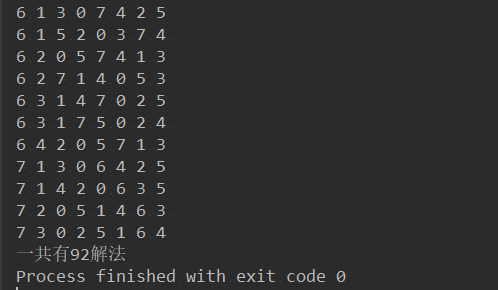a

aaa阿伟呀

2018/04/12
0
0
Java程序员进化为架构师需要掌握的知识

Java程序员进化为架构师掌握的知识： 一：Java知识 1、进制转换 2、Java基本数据类型 面向对象相关知识 3、类、接口、抽象类 this关键字、static关键字、final关键字 方法的参数传递机制 Ja...

andogo
2014/05/16
1K
3
Java虚拟机调优 JVM优化 工作非常实用的调优

JVM大体结构 class 文件 加载-验证-准备-解析-初始化-使用-卸载 一个线程一个栈，一个方法一个栈帧 如何确定垃圾 + 引用计数 会有循环引用的问题 + 正向可达 从roots对象计数可以达到的对象 ...

04/28
128
0

2011/05/12
1K
5
java 面试知识点笔记（二）底层知识 jvm 内存模型 上篇

05/14
31
0

Moks角木

2
0
VUE 计算属性

2
0

Tomcat部署的基本概念 1. CATALINA_HOME与CATALINA_BASE分别指什么？ 　　　　CATALINA_HOME指的是Tomcat的安装目录 　　　　bin：\\Tomcat一些脚本存放目录，比如启动脚本startup.bat/start...

2
0
float浮动

float浮动 float浮动概念及原理： 文档流：文档流是文档中可显示对象在排列时所占用的位置。 加浮动的元素，会脱离文档流，会沿父容器靠左或靠右排列，如果之前已经有浮动的元素，会挨着浮动...

studywin

2
0

lianbang_W

5
0